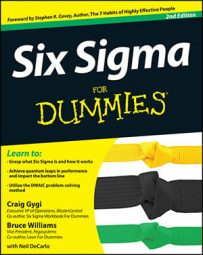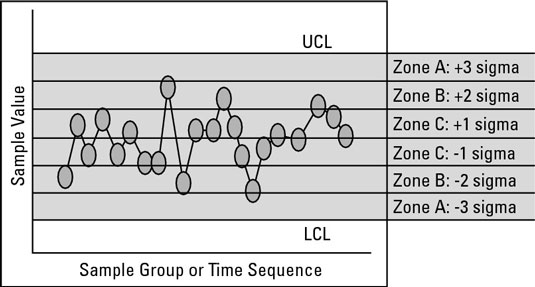##### Six Sigma For DummiesBesides control chart points that lie beyond the control limits in Six Sigma, other visual patterns can tell you that something out of the ordinary is happening to your process. These other patterns also indicate special cause variation.

Detecting special cause patterns, shifts, and drifts in a control chart is similar to detecting out-of-the-ordinary behavior in a pair of dice. The probability of rolling a 7 with two dice is 6 in 36, or about 17 percent. That’s because you have 6 possible ways to roll a 7 with two dice, out of a total of 36 possible outcomes.

What is the probability of rolling a 7 twice in a row? The combined probability is 17 percent (0.17) multiplied by 17 percent (0.17), or 2.8 percent (0.028). The probability of rolling a 7 three times in a row is 0.17 × 0.17 × 0.17, or about 0.46 percent.

So if you see someone roll a 7 three times in a row, that probability is small enough that you can safely conclude something out of the ordinary must be going on. You use this same thinking to detect patterns, trends, and shifts in control charts.

Dividing the distance between the control limits and the process average into three equal zones, you can use the following rules to detect special causes of variation:

• Any one point beyond either control limit

• Two out of any three consecutive points in Zone A, and all three on the same side of the process average

• Four out of any five consecutive points in Zone B or A, and all five on the same side of the process average

• Fifteen points in a row in Zone C, on either side of the process average